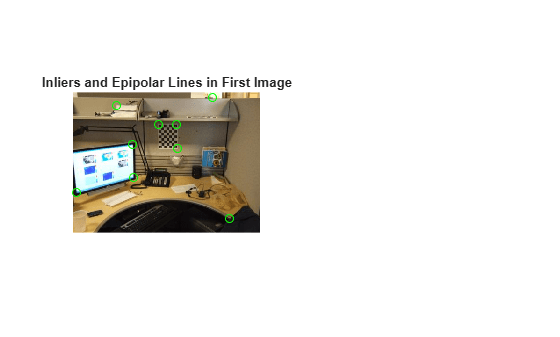# epipolarLine

Compute epipolar lines for stereo images

## Syntax

``lines = epipolarLine(F,points)``
``lines = epipolarLine(F',points)``

## Description

example

````lines = epipolarLine(F,points)` returns an M-by-3 matrix, `lines`. The matrix represents the computed epipolar lines in image `I2` corresponding to the `points` in image `I1`. The input `F` represents the fundamental matrix that maps points in `I1` to epipolar lines in image `I2`.```
````lines = epipolarLine(F',points)` The matrix represents the computed epipolar lines in image `I1` corresponding to the `points` in image `I2`.```

## Examples

collapse all

This example shows you how to compute the fundamental matrix. It uses the least median of squares method to find the inliers.

The points, matched_points1 and matched_points2, have been putatively matched.

```load stereoPointPairs [fLMedS,inliers] = estimateFundamentalMatrix(matchedPoints1,... matchedPoints2,'NumTrials',4000);```

Show the inliers in the first image.

```I1 = imread('viprectification_deskLeft.png'); figure; subplot(121); imshow(I1); title('Inliers and Epipolar Lines in First Image'); hold on; plot(matchedPoints1(inliers,1),matchedPoints1(inliers,2),'go')```Compute the epipolar lines in the first image.

`epiLines = epipolarLine(fLMedS',matchedPoints2(inliers,:));`

Compute the intersection points of the lines and the image border.

`points = lineToBorderPoints(epiLines,size(I1));`

Show the epipolar lines in the first image

`line(points(:,[1,3])',points(:,[2,4])');`Show the inliers in the second image.

```I2 = imread('viprectification_deskRight.png'); subplot(122); imshow(I2); title('Inliers and Epipolar Lines in Second Image'); hold on; plot(matchedPoints2(inliers,1),matchedPoints2(inliers,2),'go')```Compute and show the epipolar lines in the second image.

```epiLines = epipolarLine(fLMedS,matchedPoints1(inliers,:)); points = lineToBorderPoints(epiLines,size(I2)); line(points(:,[1,3])',points(:,[2,4])'); truesize;```## Input Arguments

collapse all

Fundamental matrix, specified as a 3-by-3 matrix. `F` must be double or single. If P1 represents a point in the first image I1 that corresponds to P2, a point in the second image I2, then:

 [P2,1] * `F` * [P1,1]' = 0

In computer vision, the fundamental matrix is a 3-by-3 matrix which relates corresponding points in stereo images. When two cameras view a 3-D scene from two distinct positions, there are a number of geometric relations between the 3-D points and their projections onto the 2-D images that lead to constraints between the image points. Two images of the same scene are related by epipolar geometry.

Fundamental matrix, specified as a 3-by-3 matrix. The `F'` fundamental matrix maps points in image `I2` to epipolar lines in image `I1`.

Coordinates of points, specified as an M-by-2 matrix. Each row contains the (x,y) coordinates of a point. M is the number of points.

## Output Arguments

collapse all

An M-by-3 matrix, where each row must be in the format, [A,B,C]. This corresponds to the definition of the line:

 A * x + B * y + C = 0.
M represents the number of lines.

## Version History

Introduced in R2011a